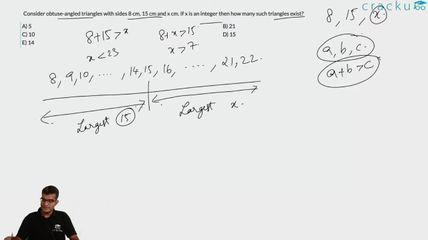Question 16

# Consider obtuse-angled triangles with sides 8 cm, 15 cm and x cm. If x is an integer then how many such triangles exist?

Solution

For obtuse-angles triangle, $$c^2 > a^2 + b^2$$ and c < a+b
If 15 is the greatest side, 8+x > 15 => x > 7 and $$225 > 64 + x^2$$ => $$x^2$$ < 161 => x <= 12
So, x = 8, 9, 10, 11, 12
If x is the greatest side, then 8 + 15 > x => x < 23
$$x^2 > 225 + 64 = 289$$ => x > 17
So, x = 18, 19, 20, 21, 22
So, the number of possibilities is 10

### View Video Solution